GET THE APP

Experimental Investigation of Energy Absorbency and Dampening Characteristics of D3O® Material during Low Velocity Static Impacts.# Journal of ErgonomicsOpen Access

Research Article - (2021)

# Experimental Investigation of Energy Absorbency and Dampening Characteristics of D3O® Material during Low Velocity Static Impacts.

Anas A. Shargawi1*, Ryan Z. Amick2, Michael J. Jorgensen1 and Ramazan Asmatulu3

*Correspondence: Anas A. Shargawi, Department of Industrial Systems and Manufacturing Engineering, Wichita State University, Kansas, USA, Email:

Author info »

## Abstract

Personal protective materials used to reduce shock impacts are available on the market for use in the sports and industry sector. Many of the materials may attenuate vibrational impact forces and protect the human body from potential injuries incurred during long term exposure. An experiment was performed to evaluate material energy absorption level, displacement effectiveness, and dampening characteristics, for two different recoil pads and one back protector D3O® materials, as well as two silicone based viscoelastic rubbery materials. Low, medium, and high energy impact levels, similar to impact forces produced by percussive power hand-held tools (i.e., rivet guns) were applied to all five samples of the materials using a low velocity impact tower to measure materials energy absorption and calculate dampening ratios. Material D3O® indicated a higher energy absorbency level (p=0.00) and a higher dampening ratio compared to silicone-based materials. However, the back-protector D3O® material indicated a much higher dampening ratio and a higher energy absorbency level compared to all other materials (p=0.00). The higher the level of energy absorbed with sufficient displacement during the impact, the higher the attenuation of transmitted impact forces through the material. This indicates which types of materials may be more appropriate to use based on the impact level. Although static testing may not represent the dynamic environment of percussive hand-held power tools, these results provide detailed information under systematic impact loads.

#### Keywords

D3O® material; Mechanical impact; Energy absorption; Recoil pads

#### Introduction

Vibrational forces produced during the use of percussive handheld power tools impact mainly the human hand, arm, and elbow. Low frequency, high impact tools, such as rivet guns may produce high impact accelerations with frequencies ranging from 20 Hz to 100 Hz . These external forces may be absorbed at the hand, arm and elbow of power tool users which may contribute to the development of various types of musculoskeletal disorders (i.e., vascular and/or neural symptoms) over the years of exposure . Sheet metal mechanics are exposed to vibratory power tools on a daily basis, which poses a potential risk of developing vibration work-related symptoms. One exposure hazard that is often present, but rarely addressed, is hand-arm vibration. Researchers explained “vibrations caused by power tools, machinery, vehicles and heavy equipment are a universal feature of modern work environments .” In USA an estimated six million workers are in occupations exposed to whole-body vibration and more than one million workers are in occupations exposed to hand-transmitted vibration .

Viscoelastic material made for vibration dampening in mechanical structures have two fundamental assumptions in the materials science and continuum mechanics literature in regards to energy absorption mechanisms. The first assumption is that the energy absorbed as a relative motion is dissipated only through the shear deformation of the viscoelastic core, for which a small amount of heat may be generated. The second assumption is that the vibration will dissipate exclusively through the compressional dampening mechanism. The majority of research studies consider the shear deformation assumption of dissipating vibration energy where the viscoelastic layers were assumed to be incompressible and the shear deformation was the only mechanism of dampening . These assumptions work well with sandwich panel materials where the elastic-viscoelastic-elastic sandwich structure is usually incompressible. Therefore, other researchers adopt the shear deformation as the only transverse displacement of the motion rather than the compressional damping mechanism in viscoelastic mechanical structures. Optimum energy absorbing materials dissipate the kinetic energy of impact loading while retaining the force on it below the damage limit. This will depend on the design of the absorbent material, such as shape, type of chemicals used in making the material, and the capacity to absorb kinetic energy .

On the market there are many different advertised materials, (e.g., viscoelastic, silicone, rubber, etc.) made for the purpose of absorbing low and high vibrational impact forces to protect the human body from such exposure that may ultimately cause workrelated symptoms and disorders. The D3O® material consists of a polyurethane with Polyborodimethylsiloxane (PBDMS), a dilatant non-Newtonian fluid that is a substance that when in its raw state is highly deformable when slowly squeezed but it hardens under a sudden shock impact. Previous studies of D3O® material provided energy absorption capabilities of resistance to force transmission and mechanical property of compression during a low velocity impact compared to many other polymers available on the market used primarily for sport wear, but these studies did not quantify an energy absorption level under different energy impact loads and did not consider the materials’ dampening characteristics [7-12]..

Although the D3O® materials appear to be materials that may potentially be utilized to reduce exposure to high impact energy exposures, little is known of the material properties that would be informative of the potential benefits for reducing such exposures. Thus, the aim of this study was to perform low impact testing to quantify D3O® materials energy absorption, displacement effectiveness, and estimate a dampening ratio compared to other silicone-based materials which are available commercially. Understanding these material properties may potentially be utilized to inform of the properties of interventions to reduce vibration exposure to humans.

#### Materials and Methods

Materials

Five elastomeric/viscoelastic materials were selected for testing, three of which were advertised to protect the human body against a sudden impact force (i.e., D3O® Rifle Harness (DRH), D3O® Recoil Pad (DRP), and D3O® Back Protector (DBP)) (Figure 1). These materials were acquired from products that are available on the market and were purchased from commercial vendors and were not samples provided by D3O® Company. Additionally, two conventional silicone materials were selected for comparison testing (i.e., Silicone Foam Pad (SFP) and Silicone Sponge Pad (SSP)) (Figure 1). These silicone materials have been used as antivibration elastomers for light duty machines.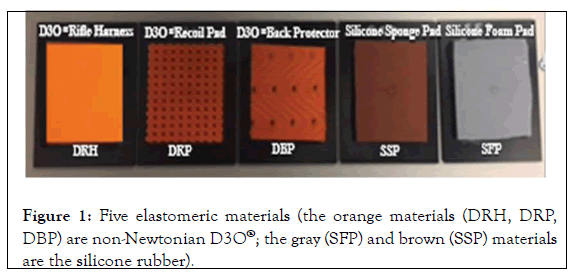Figure 1: Five elastomeric materials (the orange materials (DRH, DRP, DBP) are non-Newtonian D3O®; the gray (SFP) and brown (SSP) materials are the silicone rubber).

Study design

The dimensions for each of the five materials were 7.62 × 10.16 × 1.1 cm. Each material was glued on a 15.24 × 10.16 × 0.254 cm carbon fiber composite panel with a thin layer of adhesive GO2 (Figure 1). Each material was set up for a low velocity impact testing at three different pre-selected impact load levels using a 7.62 cm diameter tub head weighing 6.522 kg (i.e., impactor), which represented a human hand’s palm size (Figure 2). A low speed/velocity impact tower (Dynatup 8250 Drop-weight Impact Tester) was utilized to collect impact data for each of the five materials (Figure 3).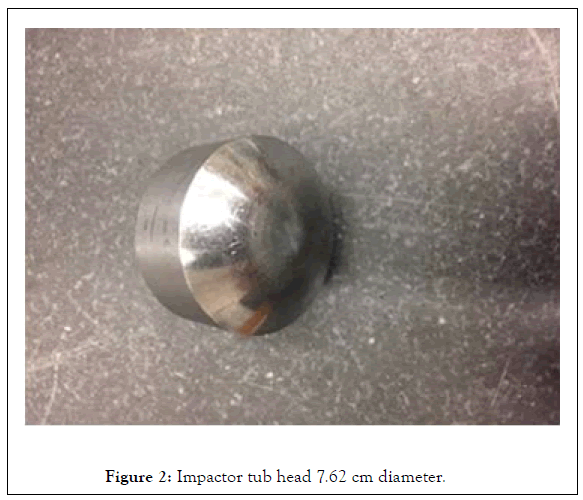Figure 2: Impactor tub head 7.62 cm diameter.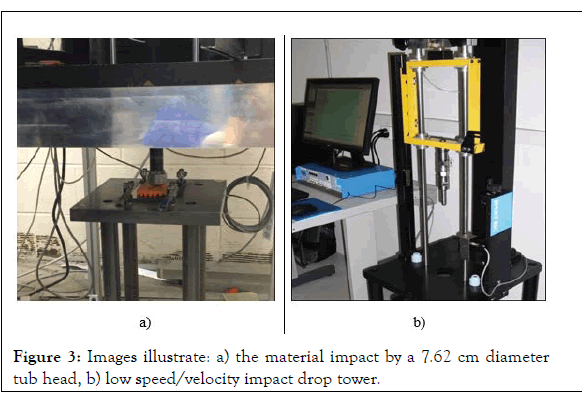Figure 3: Images illustrate: a) the material impact by a 7.62 cm diameter tub head, b) low speed/velocity impact drop tower.

Kinetic Energy (KE) equation for a falling object is given below: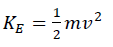(1)

Where m is the mass and v is the velocity rate. Equation (1) was utilized to set up the impact load level which was achieved by manipulating the impactor’s drop height. Each of the five materials were tested for three different drop heights, 30.90 cm, 35.33 cm, and 39.74 cm, which produced three different impact load levels: 200 kg-cm, 230 kg-cm, and 260 kg-cm, respectively. These specific impact load levels were selected to simulate the impact load produced by a percussive pneumatic aircraft rivet gun during a sheet metal riveting task, which produces vibrational forces of approximately 230 kg-cm .

Experiment protocol

Three replications were performed for each impact load level on each material under the same test conditions. The area under the resulting impact load/displacement curve was quantified utilizing the trapezoid method and the test results were used to compare between the materials mechanical properties. The area under the curve represented the amount of heat energy exchange produced by the material during the impact.

The first impact of the first replicate was set at the selected energy level of 200 kg-cm to test each of the five different materials at the specified energy level for their mechanical properties. The order of the materials being tested in the first run was DRH, DRP, DBP, SFP, and SSP. The second energy level test took place after two minutes from the first impact to test each of the same five materials at 230 kg-cm energy level. The order of the material tested for second run was SSP, SFP, DRP, DRH, and DBP. Lastly, the third energy level impact 260 kg-cm test was conducted two minutes after the second impact test to test each of the same five materials. The testing order of the material in the last run was DBP, DRH, DRP, SFP, and SSP

Each panel was impacted separately, where the time between each impact was one minute. This time was utilized for the preparation to secure the composite panel in the impact location with four locking clamps (Figure 3). The second and third replicates on each panel were performed separately on two different days, with two days between each replicate. The order of the materials tested were randomly selected for all testing conditions.

Data analysis

The amount of energy absorbed by each material, represented by the calculated area under the load/displacement curve, was quantified and analyzed. Also, the displacement level for each material was quantified and analyzed. Each material’s dampening ratio was estimated to provide a knowledge base for selecting the material that has a higher dampening ratio, which may potentially reduce the exposure of vibration.

AUC absorbed energy calculation: The Area Under the Curve (AUC) estimation utilized the trapezoids under the load/ displacement curve, which consisted of the amount of load generated versus displacement level, by drawing vertical line segments or integrals and using basic geometry calculations of each area in between each two integrals. The AUC was quantified from each replicate, and then the mean AUC of the three replicates was reported and compared between materials for each impact load.

Displacement: The displacement value was collected for each material during each impact load. The maximum displacement value for each material was recorded from each replicate, and then the mean value of the three replicates was reported and compared between materials. The displacement value was also used to calculate the dampening ratio for each material at each impact load.

Dampening ratio calculation: Data from the impact test were also used to obtain the material dampening ratio for two reasons: first, to objectively compare between materials in terms of its ability to absorb the energy by looking at the dampening mechanism, and second, to evaluate the consistency of AUC values that were previously obtained, analyzed, and compared. Maximum displacement (Xmax) of each material during each impact, angular frequency (ω), resonant frequency (ωn) spring constant (k), and force (F) were the five output data variables used to calculate the impact dampening ratio (ζ) using equation (2) for each material under each impact condition.

Dampening ratio is the damping coefficient (e.g., bouncing level of an object during an impact) divided by the critical damping coefficient (i.e., how fast the oscillation goes back to zero). The equation used to calculate the dampening ratio is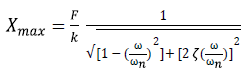(2)

Where X max is the maximum displacement, F is the force, k is the spring constant or the material stiffness level, ω is the angular frequency, ωn is the resonant frequency (also called the natural frequency), and ζ is the vibration dampening ratio (this approach solved for ζ). Variables such as force and maximum displacement were available as a result of the impact test. Variables such as spring constant, angular frequency, and resonant frequency were calculated (see equations 3 to 7 below) to obtain the dampening ratio for each material.

According to Hooke’s law, spring constant k can be calculated as the ratio of the force exerted to deform an object . Materials under impact testing were assumed to have the properties of a spring damper. The equation used to calculate the spring constant k is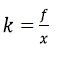(3)

Where f is the force and x is the displacement. The force f as a function of equation (3) is the compression of a spring to deform it. This is not the case for the force generated from the static single impact test using the low velocity impact tower. The force was calculated using the kinetic energy equation (1) divided by the distance travel. Therefore, equation (3) was modified to account for the impactor’s falling distance. The modified equation may be rewritten as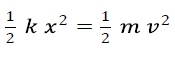(4)

Which is the same as the spring constant equation (3) but with consideration to the kinetic energy that was produced during falling. In other words, equation (4) was produced after the kinetic energy equation (1) was added to both sides of the spring constant k equation (3), which may be simplified according to equation (4) as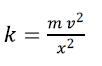(5).

Which was used to obtain the spring constant of each material for each different falling height at each energy impact level.

Angular frequency ω, also known as radial or circular frequency, measures angular displacement per unit time. Its units are therefore degrees (or radians) per second. Hence, 1 Hz ≈ 6.28 rad/sec where the angular frequency of a vibratory object may be approximated using 2π . The angular frequency in radians per second was estimated using the impact wave and the impact total time t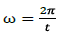(6)

The equation used to calculate the natural frequency is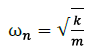Which was calculated using the square root of the spring constant k divided by mass m and was measured in radians per second. Finally, by using equation (2), the dampening ratio ζ was obtained for each material at each impact load.

Statistical analysis

A 3 × 5 two-way Analysis of Variance (ANOVA) was performed to statistically compare the difference between materials. The independent variables were energy impact level (200 kg-cm, 230 kg-cm, 260 kg-cm) and material type (DRH, DRP, DBP, SFP, SSP). The dependent variables were the amount of energy absorbed (kgf) by the materials, which was quantified by the Area Under the Curve (AUC) quantified by the trapezoid method (kgf), and the maximum displacement value (cm), which was the measured depth reached for each material during each impact load.

Post-hoc Tukey HSD tests were performed to evaluate where the differences occurred between groups after the overall statistically significant difference was determined between groups. Pairwise comparisons results were obtained with a Bonferroni adjustment. The interaction between material type and impact load effect on the energy absorption amount was analyzed. Bonferroni adjustments were made to the level of significance to reduce the probability of a type I error due to multiple comparisons. This was performed by dividing type I error that was used for the overall analysis, 0.05, by the number of comparisons that were performed (15 tests). All statistical analyses were performed by 2017 IBM® SPSS® statistics software package version .

#### Results

Most material energy absorption

The AUC was measured to represent the amount of energy absorbed by each material during the low velocity impact testing. The AUC was obtained for each of the five materials during three different impact load levels for each replicate. D3O® materials (DRH, DRP, DBP) indicated a higher amount of mean energy absorbed during impact load compared to the traditional silicone pads when tested under the same impact test conditions (Table 1).

*Energy Absorbed Area Under the Curve
Impact Load / Material Type DRH DRP DBP SFP SSP
200 Kg-cm 112.88 (1.12) 115.32 (1.15) 166.34 (0.92) 92.51 (1.13) 91.54 (1.86)
230 Kg-cm 122.93 (0.06) 128.92 (0.97) 190.47 (0.17) 94.31 (1.05) 93.8 (0.12)
260 Kg-cm 135.47 (0.47) 148.36 (0.13) 210.31 (0.22) 103.25 (0.29) 101.68 (0.46)

Table 1: Mean (standard deviation) energy absorption (kgf) as a fraction of the material type and impact load level.

Figure 4 illustrates a comparison of energy absorption and displacement level within and between materials tested. Each material absorbed more energy with more displacement level as the load of the impact increased. The DBP material absorbed more energy compared to all materials, which is shown in Table 1 by viewing the data pertaining to the amount of energy absorbed. The two-way ANOVA performed for AUC values indicated that the difference between the material types and impact load levels had a statistically significant interaction effect on the absorbed amount of energy (F (8,45)=228.792, p=0.00) (Table 2 and Figure 5).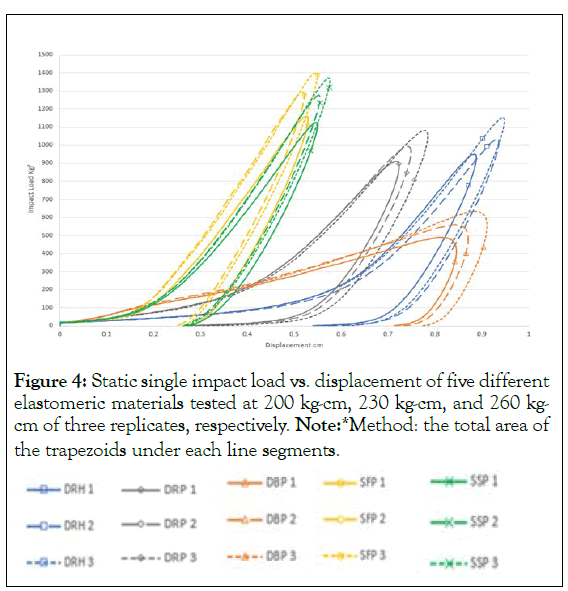Figure 4: Static single impact load vs. displacement of five different elastomeric materials tested at 200 kg-cm, 230 kg-cm, and 260 kgcm of three replicates, respectively. Note:*Method: the total area of the trapezoids under each line segments.Source SS DF MS F pvalue
Impact load 4383.63 2 2191.82 3043.01 0.00
Material type 51967.69 4 12991.92 18037.32 0.00
Impact load * Material type 1318.35 8 164.80 228.80 0.00
Error 21.61 30 0.72 - -
Total 785834.96 45 - - -

Table 2: Two-way ANOVA for material type and impact load level effect on energy absorption amount.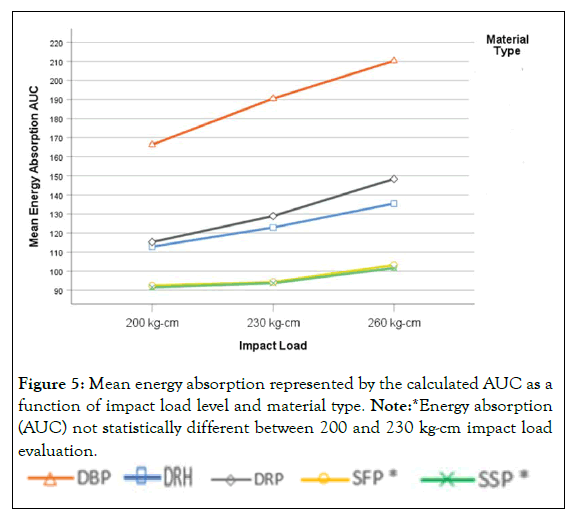Figure 5: Mean energy absorption represented by the calculated AUC as a function of impact load level and material type. Note:*Energy absorption (AUC) not statistically different between 200 and 230 kg-cm impact load evaluation.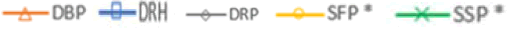Post hoc pairwise comparisons of the interaction effect showed that each material had a statistically significantly different amount of energy absorbed under different impact load level (p=0.00), except for the material SFP and SSP between impact load levels of 200 and 230 kg-cm; there was no statistically significant difference on the amount of energy absorbed, p=0.014, α=0.010. A Bonferroni adjustment was made on the overall α level for the number of comparisons (N=15) to adjust for the probability of type I error. Post hoc pairwise comparisons also indicated that material types were statistically significantly different from one another in the amount of energy absorbed across all the test conditions for low, medium, and high impacts except for silicone pads. There was no statistically significant difference between SSP and SFP across all three different energy impact levels (p=0.114) (Figure 6).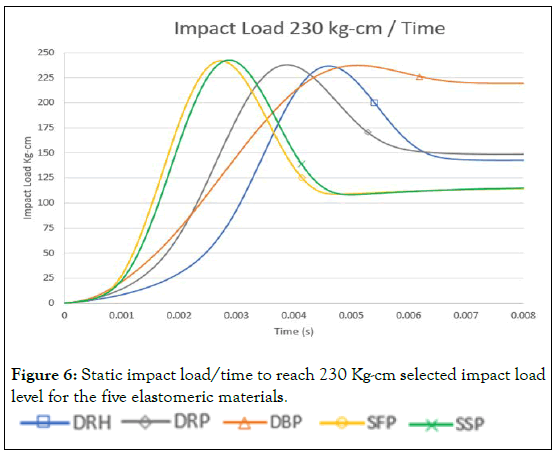Figure 6: Static impact load/time to reach 230 Kg-cm selected impact load level for the five elastomeric materials.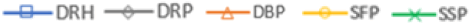The loading impact of the five elastomeric materials under the same test condition. The impact loading for each material varied in time before reaching the selected impact load level. Material D3O® consumed more impact time before reaching each selected impact load level compared to silicone rubber materials, in which that a time delay during an impact may be critically important for the absorption of the kinetic energy and dissipation into a heat.

Material displacement

As shown in Figure 7, the impact test results showed that the D3O® materials provided a greater displacement level when compared to the conventional silicone type elastomers. Silicone-based material showed a similar displacement level when the impact load increased from 200 to 230 kg-cm compared to D3O® material, which indicated a proportional increase in displacement level as the impact load increased. Two-way ANOVA was performed for the displacement level and indicated that the difference between the material type and impact load level had a statistically significant interaction effect on the displacement level (F (8,45)=15.558, p=0.00) (Table 3 and Figure 7).

Source SS DF MS F pvalue
Impact Load 0.02 2 0.01 295.79 0.00
Material type 1.14 4 0.26 11005.01 0.00
Impact load *Material type 0.003 8 0.00 15.56 0.00
Error 0.001 30 2.59E-5 - -
Total 25.14 45 - - -

Table 3: Two-way ANOVA for material type and impact load level on displacement.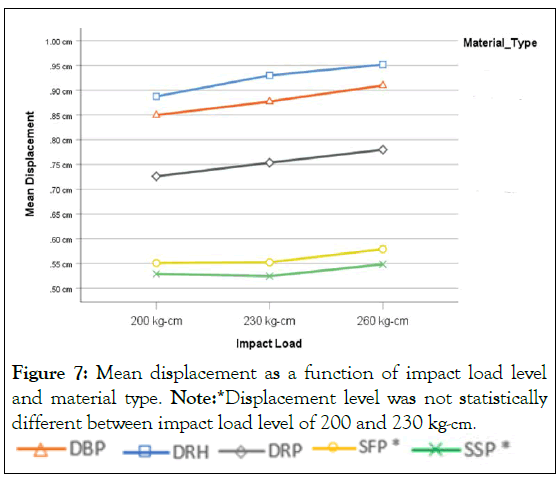Figure 7: Mean displacement as a function of impact load level and material type. Note:*Displacement level was not statistically different between impact load level of 200 and 230 kg-cm.A post hoc pairwise comparison of the interaction effect showed that each material had a statistically significantly different displacement level under different impact load level, p=0.00, except for materials SFP (p=0.296) and SSP (p=0.732) between impact load levels of 200 and 230 kg-cm, there was no statistically significant difference for the displacement level. A Bonferroni adjustment was made on the overall α level for the frequency pairwise comparisons (N=15) to adjust for the probability of type I error. Tukey HSD comparisons indicated that all material types were statistically and significantly different from one another in the amount of displacement across all impact load levels (200 kg-cm, 230 kg-cm, and 260 kg-cm), (p=0.00).

Material dampening ratio

Table 4 provides the mean dampening ratio from the static impact test. The dampening ratio results reported below indicated that DBP material had a higher dampening ratio in absorbing energy during the impact load test in comparison to the other materials.

Material Type Dampening Ratio ( ϛ )
DBP 0.16
DRH 0.10
DRP 0.11
SFP 0.05
SSP 0.05

Table 4: Mean dampening ratio from the static impact test.

DBP, DRH, and DRP dampened approximately 16%, 10%, and 11% of the impact load, respectively. SFP and SSP dampened only 5% of the impact load, which is considerably lower than D3O® materials.

#### Discussion

Material science testing studies indicate that rubber-like materials have a dampening ratio range from 0.04 to 0.07, which is an amount of energy being absorbed in a dimensionless unit [17,18]. Silicone rubber materials, SFP and SSP, showed similar values of dampening ratios that were observed during this low velocity impact testing, which can be considered a lower dampening ratio compared to D3O® materials’ dampening ratio (Table 4).

Energy absorption test results may suggest that the greater the energy absorption, the greater the dampening ratio, and the less vibrational forces transmission through protective materials into human hand-arm system. D3O® materials showed greater mechanical abilities in reducing the transmission of impact load than silicone elastomers consistently during all testing replications, which was represented in the calculated AUC (Table 1). These results are also consistent with previous studies of D3O® materials’ mechanical abilities in absorbing greater amount of energy during an impact load when compared to rubber-like materials [7-12].

The statistically significant difference that was shown in Table 2 and the quantified amount of energy being absorbed that was reported in Table 1 suggest that D3O® elastomers, when compared to other rubber-like materials available on the market, are superior at absorbing energy during an impact load; however, these results are applicable only for a static impact testing and may vary under a dynamic impact testing. The results also suggest that the five materials can be ranked in terms of greater energy absorption during an impact load as follow: 1) DBP, 2) DRP, 3) DRH, and 4) SFP and SSP. At the same time, the five materials can also be ranked for greater displacement level as follow: 1) DRH, 2) DBP, 3) DRP, 4) SSP, and 5) SFP.

DRH material displaced more load at each impact load compared to DBP, which may be explained by the locking or hardening mechanism that DBP material may acquire. The softer the material the more penetration during an impact load, but not necessarily more dampening of the vibrational waves caused by the impact [19,20]. This is true for material DRH, which displaced more load than DBP and absorbed less energy of the impact load compared to material DBP. This may explain that materials that contain air at its molecular level may act like dampers if the motion can be controlled relative to the impact [21-23]. Also, the penetrant may move from one part of the system to another as results of a random molecular motion [24,25].

Additionally, DRH material may contain air bubbles at its molecular level that depressurized faster, which may have resulted in greater displacement levels compared to DBP, which rapidly hardened during the impact allowing less penetration through its body. An important observation of DBP material was the slower penetration time through its body until reaching the selected impact load (5 millisecond) and the maintenance when reaching the selected impact load for the remaining impact time (3 millisecond) (Figure 6). This may indicate that DBP material acted as a damper and dissipated energy during the entire impact loading time compared Dilatant based material has a thickening feature where it deforms easily when it is squeezed and thickens during a sudden impact [7-10,12], and this is observed via scanning the internal structure of D30® materials during an impact . The steep line during the loading impact may show that D3O® material possess a thickening mechanism (Figure 6). The thickening feature that D3O® material possesses may allow a material to dissipate an impact load for the entire impact time by a cycle of thickening and releasing.

The shape of a curve during an impact load contributes to determining the softness-stiffness state of a material. Steep curves during an impact may indicate that a material possesses a stiff property, which may suggest ranking the materials from softer to stiffer as follow: 1) DRH, 2) DBP, 3) DRP, 4) SSP and 5) SFP. Material softness determination may also be correlated with the amount of displacement that the material offers during deformation. Furthermore, DBP material’s impact loading curve crossed the line with material DRP and DRH. This may indicate that D3O® material has a thickening-softening feature (Figure 6).

The low velocity impact test was performed under the assumption that the D3O® material has a spring damper mechanism property in accordance with Hooke’s law. This was assumed during the calculation of the dampening ratio. A compression force needed to displace a spring for a certain distance has a linear relationship with the displaced distance. Material DBP and DRP showed a nonlinear relationship in the displacement as the force increased, which indicated a different k spring constant value unlike material DRH that had a linear force displacement relationship for all impact load levels. Material type, thickness, chemical composition, compression force, and impactor shape are some factors that contribute to a constant spring value of a material during an impact force.

In this study, increasing impact load showed that material DRH possesses a low level of viscosity between medium and high impact loads represented by the non-linear relationship between the force applied and displacement level (Figure 7). The linear viscoelastic materials tend to have a k spring constant function which aids in the high predictability and accuracy during dampening ratio calculation. On another hand, materials would have a lower viscoelasticity when exposed to an extreme impact force that requires a larger deformation, which causes the impact response function to become not discrete resulting in a non-linear relationship [14,26]. Additionally, it was also assumed that the human hand tissues operate like a mass-spring-damper or viscoelastic like material that may allow its tissue to dampen small oscillations produced by high frequency, low impact tools, such as sanders . Another assumption of this experiment was the size of the impactor, 7.62 cm diameter, used to represent a hand palm size.Limitations for this study include that testing was performed at room temperature. Higher or lower temperature may affect the response of a material during an impact. Also, durability, reliability, and biocompatibility were not addressed in this study. During testing, the D3O® material was at a flat orientation during the impact, so it is unknown if the material’s response will be identical when wrapped around a handheld power tool handle

#### Conclusion

Five different elastomeric materials were tested for the energy absorption and impact dampening. The conventional silicone rubber elastomers showed statistically significantly less energy absorption when compared to the non-Newtonian D3O® materials (DBP, DRH, DRP). DBP material shows superiority in energy absorption; there was also a statistically significant difference when compared to DRH and DRP in the amount of energy absorbed at the impact. D3O® material may effectively deform and be responsive to different impact load levels, thus the silicone material may be less responsive to the lower impact force. Besides the amount of energy absorbed, the mechanical characteristics of how energy is absorbed by a material are crucial. The results suggested that some material may acquire a multiple feature such as thickening and softening, DBP may possess a stiffness-softness feature that allow DBP to uniquely dampen the impact loading. It is recommended to conduct a dynamic impact testing to further evaluate the energy absorbency and mechanical dampening abilities of D3O® materials and other possible candidates. At the same time, the further testing allows results comparison of static single impact to the dynamic cyclic impacts. This study may potentially influence the application of materials for sheet metal operators to reduce vibrational forces exposure in different manufacturing industries.

#### Acknowledgments

The authors greatly acknowledge the National Institute of Aviation Research at Wichita State University for technical support of the study.

#### Conflict of Interest Statement

This research did not receive any specific grant from funding agencies in the public, commercial, or not-for-profit sectors.

#### Author Info

Anas A. Shargawi1*, Ryan Z. Amick2, Michael J. Jorgensen1 and Ramazan Asmatulu3

1Department of Industrial Systems and Manufacturing Engineering, Wichita State University, Kansas, USA
2College of Innovation and Design, Wichita State University, Kansas, USA
3Department of Mechanical Engineering, Wichita State University, Kansas, USA

Citation: Shargawi AA, Amick RZ, Jorgensen MJ, Asmatulu R (2021) Experimental Investigation of Energy Absorbency and Dampening Characteristics of D3O® Material during Low Velocity Static Impacts. J Ergonomics. S5:003.

Received: 18-Oct-2021 Published: 08-Nov-2021, DOI: 10.35248/2165-7556.21.s5.003

Copyright: ©2021 Shargawi AA, et al. This is an open-access article distributed under the terms of the Creative Commons Attribution License, which permits unrestricted use, distribution, and reproduction in any medium, provided the original author and source are credited.

Top